# 1 Introduction

## 1.1 Rationale for the package

There has been a long relationship between the disciplines of Epidemiology / Public Health and Biostatistics. Students frequently find introductory textbooks explaining statistical methods and the maths behind them, but how to implement those techniques in a computer is most of the time not explained.

One of the most popular statistical software’s in public health settings is Stata. Stata has the advantage of offering a solid interface with functions that can be accessed via the use of commands or by selecting the proper input in the graphical unit interface (GUI). Furthermore, Stata offers “Grad Plans” to postgraduate students, making it relatively affordable from an economic point of view.

R is a free alternative to Stata. The use of R keeps growing; furthermore, with the relatively high number of packages and textbooks available, it’s popularity is also increasing.

In the case of epidemiology, there are already some good packages available for R, including: Epi, epibasix, epiDisplay, epiR and epitools. The pubh package does not intend to replace any of them, but to only provide a common syntax for the most frequent statistical analysis in epidemiology.

## 1.2 Syntax: the use of formulas

Most students and professionals from the disciplines of Epidemiology and Public Health analyse variables in terms of outcome, exposure and confounders. The following table shows the most common names used in the literature to characterise variables in a cause-effect relationships:

Response variable Explanatory variable(s)
Outcome Exposure and confounders
Outcome Predictors
Dependent variable Independent variable(s)
y x

In R, formulas are used to declare relationships between variables. Formulas are common in classic statistical tests and in regression methods.

Formulas have the following standard syntax:

y ~ x, data = my_data

Where y is the outcome or response variable, x is the exposure or predictor of interest and my_data specifies the name of the data frame where x and y can be found.

The symbol ~ is used in R for formulas. It can be interpreted as depends on. In the most typical scenario, y~x means y depends on x or y is a function of x:

y = f(x)

Using epidemiology friendly terms:

Outcome = f(Exposure)

Is worth to mention that Stata requires for variables to be given in the same order as in formulas: outcome first, predictors next.

### 1.2.1 Stratification

One way to control for confounders is the use of stratification. In Stata stratification is done by including the by option as part of the command. In the lattice package, one way of doing stratification is with a formula like:

y ~ x|z, data = my_data

Where y is the outcome or response variable, x is the exposure or predictor of interest, z is the stratification variable (a factor) and my_data specifies the name of the data frame where x, y and z can be found.

## 1.3 Contributions of the pubh package

The main contributions of the pubh package to students and professionals from the disciplines of Epidemiology and Public Health are:

1. Use of a common syntax for the most used analysis.
2. A function, glm_coef that displays coefficients from most common regression analysis in a way that can be easy interpreted and used for publications.
3. Integration with the lattice package, introducing plotting functions with a relatively simple syntax.
4. Integration with the most common epidemiological analysis, using the standard formula syntax explained in the previous section.

# 2 Descriptive statistics

Many options currently exists for displaying descriptive statistics. I recommend the function summarise from the papeR package. It does a great job for both continuous and categorical (factors) outcomes.

The estat function from the pubh package displays descriptive statistics of continuous outcomes and like summarise, it can use labels to display nice tables. estat has less options than summarise and as such, is simpler to use, which is the main intention of the pubh package. Furthermore, as a way to aid in the understanding of the variability, estat displays also the relative dispersion (coefficient of variation) which is of particular interest for comparing variance between groups (factors).

Some examples. We start by loading packages as suggested in the Template of this package.

library(effects, warn.conflicts = FALSE)
library(latex2exp, warn.conflicts = FALSE)
library(multcomp, warn.conflicts = FALSE)
library(pander, warn.conflicts = FALSE)
library(papeR, warn.conflicts = FALSE)
library(pubh, warn.conflicts = FALSE)

panderOptions("table.split.table", Inf)
set.alignment("right", row.names = "left", permanent = TRUE)
trellis.par.set(tactile.theme())

We will use a data set about blood counts of T cells from patients in remission from Hodgkin’s disease or in remission from disseminated malignancies.

data(Hodgkin)
Hodgkin$Ratio <- Hodgkin$CD4/Hodgkin$CD8 pander(head(Hodgkin)) CD4 CD8 Group Ratio 396 836 Hodgkin 0.4737 568 978 Hodgkin 0.5808 1212 1678 Hodgkin 0.7223 171 212 Hodgkin 0.8066 554 670 Hodgkin 0.8269 1104 1335 Hodgkin 0.827 Descriptive statistics for CD4+ T cells: estat(~ CD4, data = Hodgkin) #> N Min. Max. Mean Median SD CV #> 1 CD4 40 116 2415 672.62 528.5 470.49 0.7 In the previous code, the left-hand side of the formula is empty as it’s the case when working with only one variable. We can use pander to display nice tables with labels. pander(estat(~ CD4, data = Hodgkin, label = "CD4^+^ T cells (cells / mm^3^)")) N Min. Max. Mean Median SD CV CD4+ T cells (cells / mm3) 40 116 2415 672.6 528.5 470.5 0.7 In the previous command, we used the Markup language for the superscripts in the label. For subscripts, the syntax is (for example): H~2~O. For stratification, estat recognises the following two syntaxes: • outcome ~ categorical predictor • ~ outcome | categorical predictor For example: pander(estat(~ Ratio|Group, data = Hodgkin, label = "CD4^+^/CD8^+^ T cells")) Group N Min. Max. Mean Median SD CV CD4+/CD8+ T cells Non-Hodgkin 20 1.1 3.49 2.12 2.15 0.73 0.34 Hodgkin 20 0.47 3.82 1.5 1.19 0.91 0.61 ## 2.1 Inferential statistics From the descriptive statistics of Ratio we know that the relative dispersion in the Hodgkin group is almost as double as the relative dispersion in the Non-Hodgkin group. For new users of R it helps to have a common syntax in most of the commands, as R could be challenging and even intimidating at times. We can test if the difference in variance is statistically significant with the var.test command, which uses can use a formula syntax: var.test(Ratio ~ Group, data = Hodgkin) #> #> F test to compare two variances #> #> data: Ratio by Group #> F = 0.6294, num df = 19, denom df = 19, p-value = 0.3214 #> alternative hypothesis: true ratio of variances is not equal to 1 #> 95 percent confidence interval: #> 0.2491238 1.5901459 #> sample estimates: #> ratio of variances #> 0.6293991 What about normality? We can look at the QQ-plots against the standard Normal distribution. lab <- TeX("CD4$^+$/ CD8$^+$T cells") qq_plot(~ Ratio|Group, data = Hodgkin, ylab = lab)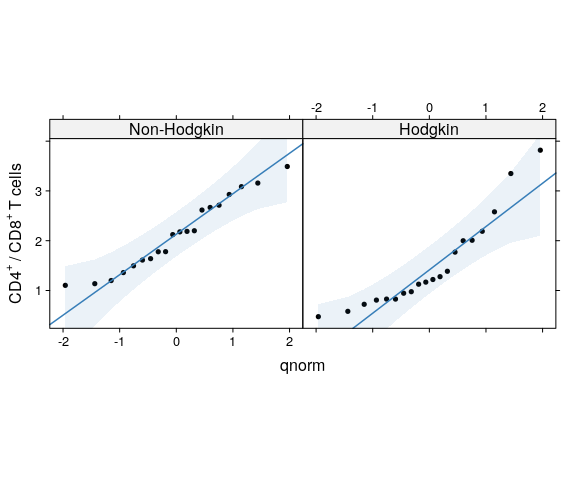Let’s say we choose a non-parametric test to compare the groups: wilcox.test(Ratio ~ Group, data = Hodgkin) #> #> Wilcoxon rank sum test #> #> data: Ratio by Group #> W = 298, p-value = 0.007331 #> alternative hypothesis: true location shift is not equal to 0 ## 2.2 Graphical output For relatively small samples (for example, less than 30 observations per group) is a standard practice to show the actual data in dot plots with error bars. The pubh package offers two options to show graphically differences in continuous outcomes among groups: • For small samples: strip_error • For medium to large samples: bar_error For our current example: strip_error(Ratio ~ Group, data = Hodgkin, ylab = lab)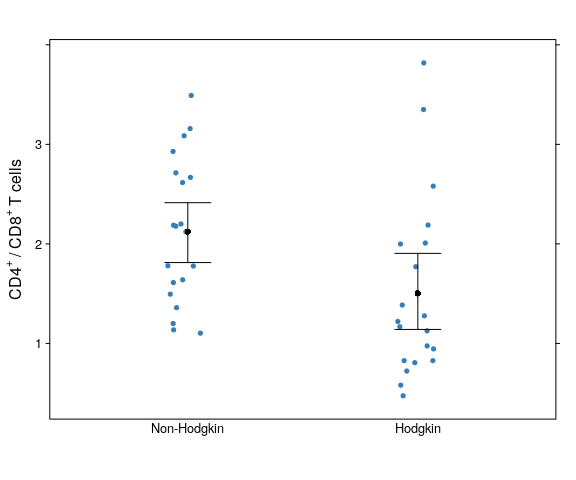The error bars represent 95% confidence intervals around mean values. Is relatively easy to add a line on top, to show that the two groups are significantly different. With the latticeExtra package (loaded by default with pubh) allows the use of layers. We will draw a line using panel.segments and text with panel.text. For panel.segments we provide the coordinates of the two points at the ends of the line in the following order: x1, y1, x2, y2. x coordinates are easy: from 1 to 2. For the y coordinates, we choose a value higher than the maximum observation (from our descriptives, higher than 3.82). We also need to adjust the limits of the y-axis to accommodate for our line and text. It could be some trial and error one has the plot that is more visually appealing. The commands are as follows: strip_error(Ratio ~ Group, data = Hodgkin, ylab = lab, ylim = c(0, 4.5)) + layer(panel.segments(1, 4, 2, 4, lwd=1.5)) + layer(panel.text(1.5, 4.1, "**"))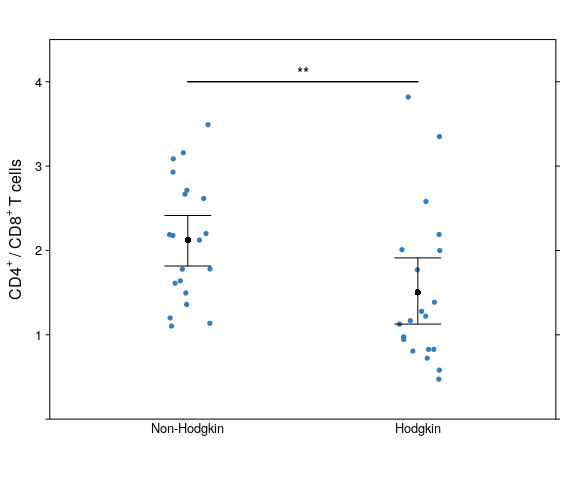For larger samples we would use bar charts with error bars. For example: data(birthwt) birthwt$smoke <- factor(birthwt$smoke, labels = c("Non-smoker", "Smoker")) birthwt$race <- factor(birthwt$race, labels = c("White", "African American", "Other")) Bar charts should always start at the origin, the upper limit on the $$y$$ scale should be higher than the maximum upper confidence limit. For example: pander(gen_bst_df(bwt ~ smoke, data = birthwt)) bwt LowerCI UpperCI smoke 3056 2923 3186 Non-smoker 2772 2616 2910 Smoker bar_error(bwt ~ smoke, data = birthwt, ylab = "Birth weight (g)", ylim = c(0, 3500))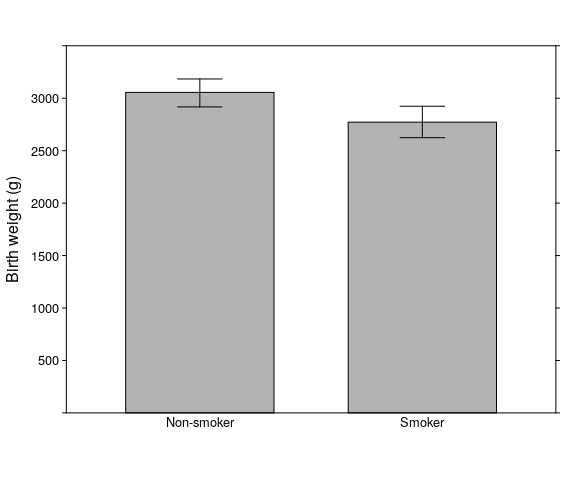Quick normality check: qq_plot(~ bwt|smoke, data = birthwt, ylab = "Birth weight (g)")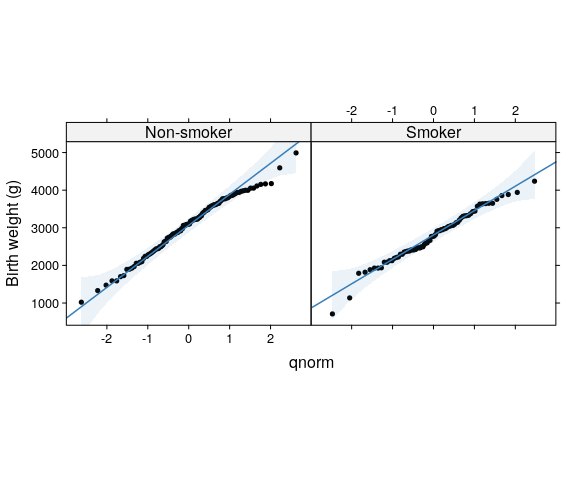The (unadjusted) test: t.test(bwt ~ smoke, data = birthwt) #> #> Welch Two Sample t-test #> #> data: bwt by smoke #> t = 2.7299, df = 170.1, p-value = 0.007003 #> alternative hypothesis: true difference in means is not equal to 0 #> 95 percent confidence interval: #> 78.57486 488.97860 #> sample estimates: #> mean in group Non-smoker mean in group Smoker #> 3055.696 2771.919 The final plot showing (unadjusted) difference: bar_error(bwt ~ smoke, data = birthwt, ylab = "Birth weight (g)", ylim = c(0, 3500)) + layer(panel.segments(1, 3300, 2, 3300, lwd=1.5)) + layer(panel.text(1.5, 3350, "**"))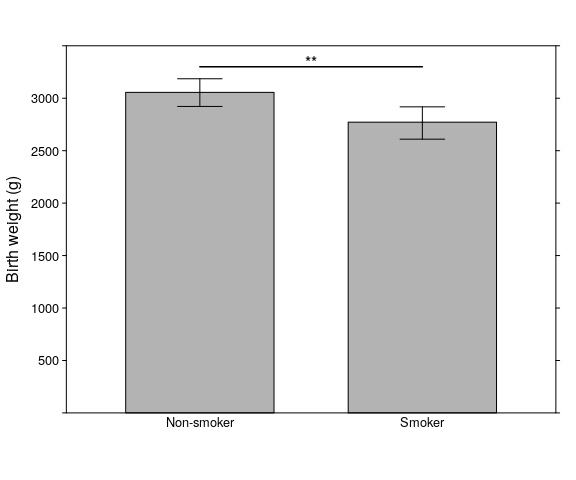Both strip_error and bar_error can generate plots stratified by a third variable, for example: bar_error(bwt ~ smoke|race, data = birthwt, ylab = "Birth weight (g)", ylim = c(0, 3800), col = c("gray80", "gray30"))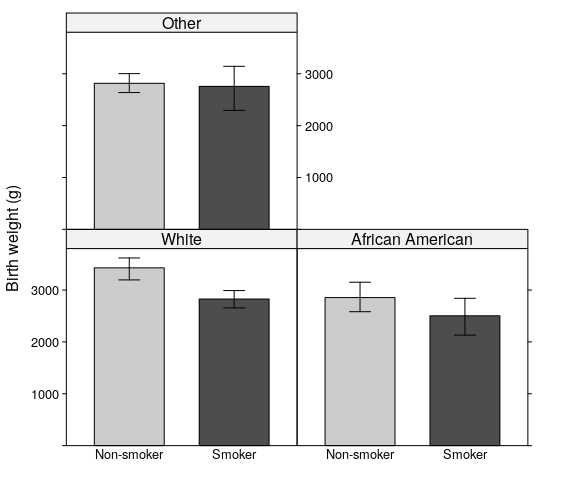strip_error(bwt ~ smoke|race, data = birthwt, ylab = "Birth weight (g)", cex = 0.3)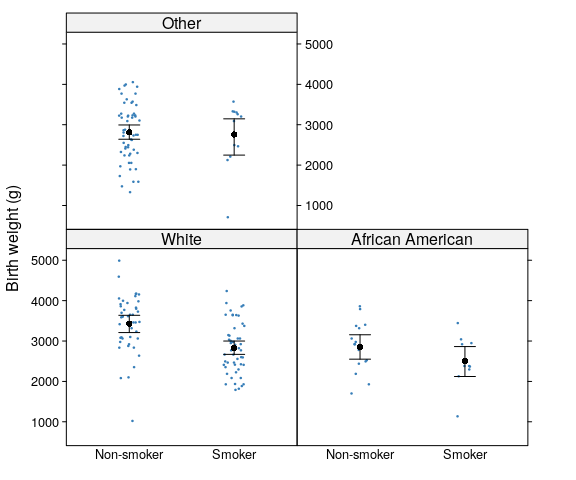# 3 Regression models The pubh package includes the function glm_coef for displaying coefficients from regression models and the function xymultiple to help in the visualisation of multiple comparisons. Note: Please read the vignette on Regression Examples for a more comprehensive use of glm_coef. For simplicity, here we show the analysis of the linear model of smoking on birth weight, adjusting by race (and not by other potential confounders). model_bwt <- lm(bwt ~ smoke + race, data = birthwt) glm_coef(model_bwt) #> Coefficient Pr(>|t|) #> (Intercept) 3334.95 (3036.11, 3633.78) < 0.001 #> smokeSmoker -428.73 (-667.02, -190.44) < 0.001 #> raceAfrican American -450.36 (-750.31, -150.4) 0.003 #> raceOther -452.88 (-775.17, -130.58) 0.006 unadj <- glm_coef(model_bwt, labels = c("Constant", "Smoking: smoker - non-smoker", "Race: African American - White", "Race: Other - White")) pander(unadj) Coefficient Pr(>|t|) Constant 3334.95 (3036.11, 3633.78) < 0.001 Smoking: smoker - non-smoker -428.73 (-667.02, -190.44) < 0.001 Race: African American - White -450.36 (-750.31, -150.4) 0.003 Race: Other - White -452.88 (-775.17, -130.58) 0.006 In the previous table of coefficients confidence intervals and p-values for race had not been adjusted for multiple comparisons. We use functions from the multcomp package to make the corrections. model_glht <- glht(model_bwt, linfct = mcp(race = "Tukey")) xymultiple(model_glht) #> Comparison Difference lwr upr Pr(>|Z|) #> 1 African American - White -450.36 -810.70 -90.02 0.011 #> 2 Other - White -452.88 -726.99 -178.77 < 0.001 #> 3 Other - African American -2.52 -380.45 375.41 1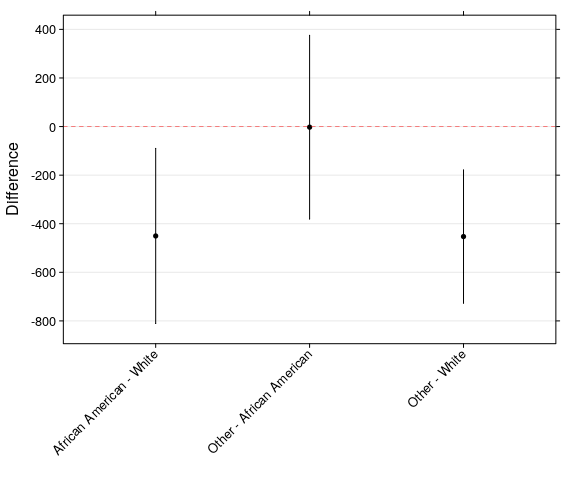We can incorporate the adjusted results into our final table: race_glht <- xymultiple(model_glht, plot = FALSE) pander(race_glht) Comparison Difference lwr upr Pr(>|Z|) African American - White -450.4 -810.8 -89.97 0.011 Other - White -452.9 -727 -178.7 < 0.001 Other - African American -2.52 -380.5 375.5 1 final <- unadj final[, 1] <- as.character(final[, 1]) final[3:4, 1] <- paste0(race_glht[1:2, 2], " (", race_glht[1:2, 3], ", ", race_glht[1:2, 4], ")") final[, 2] <- as.character(final[, 2]) final[3:4, 2] <- as.character(race_glht[1:2, 5]) pander(final) Coefficient Pr(>|t|) Constant 3334.95 (3036.11, 3633.78) < 0.001 Smoking: smoker - non-smoker -428.73 (-667.02, -190.44) < 0.001 Race: African American - White -450.36 (-810.75, -89.97) 0.011 Race: Other - White -452.88 (-727.02, -178.73) < 0.001 The effect plot: plot(Effect(c("race", "smoke"), model_bwt), main = NULL, aspect = 3/4, multiline = TRUE, ylab = "Birth weight (g)", xlab = "Race/Ethnicity", symbols = list(pch = 16))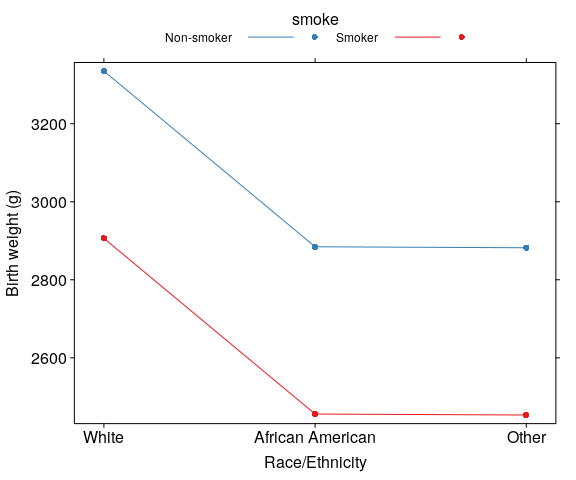# 4 Epidemiology functions The pubh package offers two wrappers to epiR functions. 1. contingency calls epi.2by2 and it’s used to analyse two by two contingency tables. 2. diag_test calls epi.tests to compute statistics related with screening tests. ## 4.1 Contingency tables Let’s say we want to look at the effect of ibuprofen on preventing death in patients with sepsis. data(Bernard) pander(head(Bernard)) id treat race fate apache o2del followup temp0 temp10 1 Placebo White Dead 27 539.2 50 35.22 36.56 2 Ibuprofen African American Alive 14 NA 720 38.67 37.56 3 Placebo African American Dead 33 551.1 33 38.33 NA 4 Ibuprofen White Alive 3 1376 720 38.33 36.44 5 Placebo White Alive 5 NA 720 38.56 37.56 6 Ibuprofen White Alive 13 1523 720 38.17 38.17 Let’s look at the table: tbl1 <- summarise(Bernard, type = "fac", variables = "treat", group = "fate", test = FALSE, labels = "Treatment") first_row <- c(rep("", 3), "Alive", rep("", 2), "Dead", "") tbl1 <- rbind(first_row, tbl1) pander(tbl1) Level N % N % Alive Dead Treatment Placebo 139 49.8 92 52.3 Ibuprofen 140 50.2 84 47.7 For epi.2by2 we need to provide the table of counts in the correct order, so we would type something like: dat <- matrix(c(84, 140 , 92, 139), nrow = 2, byrow = TRUE) epiR::epi.2by2(as.table(dat)) #> Outcome + Outcome - Total Inc risk * #> Exposed + 84 140 224 37.5 #> Exposed - 92 139 231 39.8 #> Total 176 279 455 38.7 #> Odds #> Exposed + 0.600 #> Exposed - 0.662 #> Total 0.631 #> #> Point estimates and 95 % CIs: #> ------------------------------------------------------------------- #> Inc risk ratio 0.94 (0.75, 1.19) #> Odds ratio 0.91 (0.62, 1.32) #> Attrib risk * -2.33 (-11.27, 6.62) #> Attrib risk in population * -1.15 (-8.88, 6.59) #> Attrib fraction in exposed (%) -6.20 (-33.90, 15.76) #> Attrib fraction in population (%) -2.96 (-15.01, 7.82) #> ------------------------------------------------------------------- #> X2 test statistic: 0.26 p-value: 0.61 #> Wald confidence limits #> * Outcomes per 100 population units For contingency we only need to provide the information in a formula: contingency(fate ~ treat, data = Bernard) #> Outcome #> Predictor Dead Alive #> Ibuprofen 84 140 #> Placebo 92 139 #> #> Outcome + Outcome - Total Inc risk * #> Exposed + 84 140 224 37.5 #> Exposed - 92 139 231 39.8 #> Total 176 279 455 38.7 #> Odds #> Exposed + 0.600 #> Exposed - 0.662 #> Total 0.631 #> #> Point estimates and 95 % CIs: #> ------------------------------------------------------------------- #> Inc risk ratio 0.94 (0.75, 1.19) #> Odds ratio 0.91 (0.62, 1.32) #> Attrib risk * -2.33 (-11.27, 6.62) #> Attrib risk in population * -1.15 (-8.88, 6.59) #> Attrib fraction in exposed (%) -6.20 (-33.90, 15.76) #> Attrib fraction in population (%) -2.96 (-15.01, 7.82) #> ------------------------------------------------------------------- #> X2 test statistic: 0.26 p-value: 0.61 #> Wald confidence limits #> * Outcomes per 100 population units #> #> #> Pearson's Chi-squared test with Yates' continuity correction #> #> data: dat #> X-squared = 0.17076, df = 1, p-value = 0.6794 Advantages of contingency: 1. Easier input without the need to create the table. 2. Displays the standard epidemiological table at the start of the output. This aids to check what are the reference levels on each category. 3. In the case that the $$\chi^2$$-test is not appropriate, contingency would show the results of the Fisher exact test at the end of the output. ### 4.1.1 Mantel-Haenszel odds ratio For mhor the formula has the following syntax: outcome ~ stratum/exposure, data = my_data Thus, mhor displays the odds ratio of exposure yes against exposure no on outcome yes for different levels of stratum, as well as the Mantel-Haenszel pooled odds ratio. Example: effect of eating chocolate ice cream on being ill by sex from the oswego data set. data(oswego, package = "epitools") oswego$ill <- factor(oswego$ill) oswego$sex <- factor(oswego$sex) oswego$chocolate.ice.cream <- factor(oswego\$chocolate.ice.cream)
mhor(ill ~ sex/chocolate.ice.cream, data = oswego)
#>
#>                             OR Lower.CI Upper.CI Pr(>|z|)
#> sexF:chocolate.ice.creamY 0.47     0.11     2.06    0.318
#> sexM:chocolate.ice.creamY 0.24     0.05     1.13    0.072
#>
#>                           Common OR Lower CI Upper CI Pr(>|z|)
#> Cochran-Mantel-Haenszel:       0.35     0.12     1.01    0.085
#>
#> Test for effect modification (interaction): p =  0.5434
#> 

### 4.1.2 Odds trend

Example: An international case-control study to test the hypothesis that breast cancer is related to the age that a woman gives childbirth.

odds_trend(Cancer ~ Age, data = Macmahon)
#> Odds Ratios with 95% CIs and p-values
#>
#>  Exposure   OR lower upper chi.square fisher.exact
#>       <20 1.00    NA    NA       <NA>         <NA>
#>     20-24 1.21  1.06  1.39      0.007        0.007
#>     25-29 1.55  1.35  1.79    < 0.001      < 0.001
#>     30-34 1.88  1.60  2.22    < 0.001      < 0.001
#>       >34 2.41  1.96  2.95    < 0.001      < 0.001
#>
#>
#> Chi-squared Test for Trend in Proportions = 129.012
#>  1 d.f., p < 0.001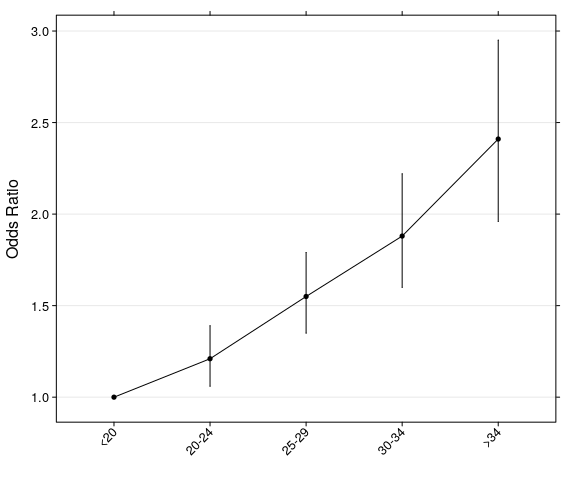## 4.2 Diagnostic tests

Example: We compare the use of lung’s X-rays on the screening of TB against the gold standard test.

Freq <- c(1739, 8, 51, 22)
BCG <- gl(2, 1, 4, labels=c("Negative", "Positive"))
Xray <- gl(2, 2, labels=c("Negative", "Positive"))
tb <- data.frame(Freq, BCG, Xray)
tb <- expand_df(tb)

diag_test(BCG ~ Xray, data=tb)
#>           Outcome +    Outcome -      Total
#> Test +           22           51         73
#> Test -            8         1739       1747
#> Total            30         1790       1820
#>
#> Point estimates and 95 % CIs:
#> ---------------------------------------------------------
#> Apparent prevalence                    0.04 (0.03, 0.05)
#> True prevalence                        0.02 (0.01, 0.02)
#> Sensitivity                            0.73 (0.54, 0.88)
#> Specificity                            0.97 (0.96, 0.98)
#> Positive predictive value              0.30 (0.20, 0.42)
#> Negative predictive value              1.00 (0.99, 1.00)
#> Positive likelihood ratio              25.74 (18.21, 36.38)
#> Negative likelihood ratio              0.27 (0.15, 0.50)
#> ---------------------------------------------------------

Using the immediate version (direct input):

diag_test2(22, 51, 8, 1739)
#>
#>           Outcome +    Outcome -      Total
#> Test +           22           51         73
#> Test -            8         1739       1747
#> Total            30         1790       1820
#>
#> Point estimates and 95 % CIs:
#> ---------------------------------------------------------
#> Apparent prevalence                    0.04 (0.03, 0.05)
#> True prevalence                        0.02 (0.01, 0.02)
#> Sensitivity                            0.73 (0.54, 0.88)
#> Specificity                            0.97 (0.96, 0.98)
#> Positive predictive value              0.30 (0.20, 0.42)
#> Negative predictive value              1.00 (0.99, 1.00)
#> Positive likelihood ratio              25.74 (18.21, 36.38)
#> Negative likelihood ratio              0.27 (0.15, 0.50)
#> ---------------------------------------------------------